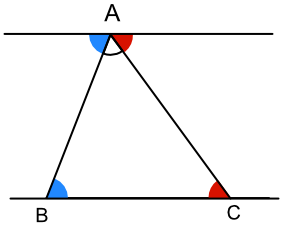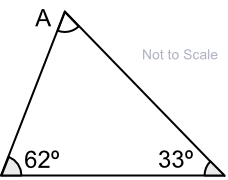Angles in a Triangle

# Angles in a Triangle

GCSE(F),

A Triangle has three sides and three angles.

The angles in a triangle always add up to 180º.

There are four types of triangle:(Note that the mark on some of the lines means that it is the same length as another line with the same mark on the diagram).

The number of degrees in a triangle can be derived using Alternate Angles:
Angles at A and B (blue) are alternate angles;
Angles at A and C (red) are alternate angles;
Angles at A (blue, white and red) are on a straight line = 180º;
Therefore the number of degrees in a triangle is 180.## Examples

1. What is the size of angle A?